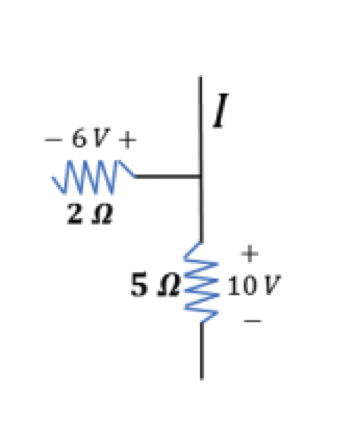# Problem: In the picture on the below, what is the current in the wire above the junction?Does charge flow toward or away from the junction?

###### FREE Expert Solution

Current:

$\overline{){\mathbf{i}}{\mathbf{=}}\frac{\mathbf{V}}{\mathbf{R}}}$

Current through the 2Ω resistor:

I1 = 6/2 = 3A

91% (220 ratings)###### Problem Details

In the picture on the below, what is the current in the wire above the junction?
Does charge flow toward or away from the junction?